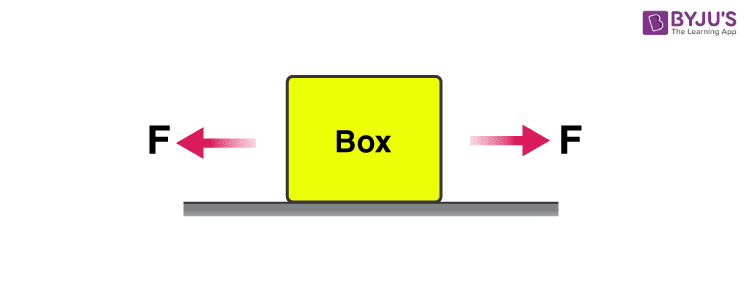# Force And Acceleration

## A Relationship Between Force and Acceleration

Newton’s laws are one of the most fundamental laws of physics. According to the first law, we know that a body will continue to be in a state of rest or uniform motion until a net external force acts on it. So what force actually is? We can define force as an interaction that changes the state of a body i.e. from rest to motion or vice-versa. But one thing to look here carefully is that the law says net force should act on the body. So we will see what that means. Consider the following situation:One force is acting towards the right and an equal force is acting towards the left hence the net force on the body is zero so the body remains at rest. So if a force is acting on a body does not mean that the state of the body will change, it may or may not. Some common forces that we experience in our daily lives are Gravitational force, frictional force, normal force, etc.

Now if a net force acts on the body then velocity either increases or decreases. This rate of change of velocity is nothing but acceleration. We also observe that the greater the force greater is this acceleration. So there must be some relation between force and acceleration and this is given by Newton’s second law. According to Newton’s second law:

F = ma

From the above relation, we can say that acceleration not only depends on the force applied but also on the mass of the body on which it is applied. If the same force is applied to two different bodies than one with a smaller mass will have greater acceleration. This is just an introduction to force and acceleration.

Following is the table explaining concepts related to force and acceleration:

Stay tuned with BYJU’S to know more about Newton’s law and how we can apply them in the world.

Test Your Knowledge On Force And Acceleration!

#### 1 Comment

1. Calum Wideboi Thomson

Very helpful thank you much better now good